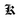JavaScript - A comedian for beginners

June 02, 2018

I always used to be serious while learning programming languages, but that did not happen with JavaScript, especially when I was learning the basics. Before I write about some amusing things about JavaScript, let me throw some light on the versatility of the JavaScript.

When it comes to web development, JavaScript is present everywhere. Frontend - ReactJS, AngularJS, etc. Backend - Express.js, Meteor.js, etc. Guess what? You can also build an Android app using your ReactJS skills, and for that, we have React Native to the rescue. Being a beginner, I am not aware of more amazing frameworks, but I admit that JavaScript is very versatile and a very interesting language to learn.

Now let’s see some fun facts - but very important to remember, to avoid coding mistakes.

The implicit type conversion for Arithmetic operators

Multiplication

The obvious way to multiply 2 by 2

2 * 2 = 4

JavaScript can also take string operands and perform implicit type conversion on them, provided they are convertible into numeric values. For example,

"5" * 2 = 10

OR

"3" * "3" = 9

"JS" * 6 = NaN  //"JS" cannot be converted to a number

"Two" * 3 = NaN //"Two" cannot be converted to a number as well

Division

The obvious way to divide 10 by 2

10 / 2 = 5

By now, you might be familiar.

"30" / 2 = 15

Again,

"100" / "4" = 25

Let’s get this done with the last operator.

Well, this needs no explanation.

5 + 5 = 10

But,

"10" + 10 = 1010

WTF? 1010? No implicit type conversion?

Yes, things work quite differently for ”+” operator, so whenever one of the operands is of type string, the other operand gets converted to the string type too.

For addition to work, both the operands should be of numeric types.

10 + 10 = 20

OR

30 + Number("20") = 50

OR

20 + +"20" = 40

What? - Wait, I am not drunk. That is an amazing thing about using ”+” as a unary operator. Prepend it to any string representing a number, and it converts the string into its equivalent numeric value.

For simplicity:

20 + (+"20") = 40

Hold on, let me show you some more interesting things about using ”+” as a unary operator. Caution: Do not go crazy.

25 + +null = 25

Also,

50 + +"" = 50

In JS, the equivalent numeric value for the null and empty string is 0.

Point to note: If you prepend it to any string containing alphabetic keywords like “Hulk” or “Tron”, it would result into NaN (Not a Number) and any operation with NaN, results as NaN.

The comparison and equality operators

Usually comparison and equality operators like >, >=, <, <=, and == are used with numbers.

For example, let’s say we want to check if 2 is greater than 1.

2 > 1

This will return a boolean value, either true or false, depending on the comparison. In above example, it will return true.

Well, you won’t be surprised to know that JavaScript performs implicit type conversion here as well - just like it did for arithmetic operators.

Let’s provide a string representing a numeric value.

"10" == 100

Returns false.

"100" > "10"

Returns true.

Let’s try out something cooler.

true > 0

Returns true, since true is represented as 1 in its numeric form, 1 > 0 is always true.

false <= 0

Returns true, since false is represented as 0 in its numeric form, 0 <= 0 is always true.

Also, for empty strings,

"" > -1

Returns true, since ”” (empty string) is represented as 0 in it’s numeric form, 0 > -1 is always true.

Same applies to null,

null > 0

Returns false, because null is represented as 0, and 0 > 0 is always false.

Also,

null >= 0

Returns true, as null is not greater than 0, from this we can confirm that null is equal to 0.

But,

null == 0

Returns false.

WTF? If null > 0 is false, and null >= 0 is true, it becomes clear that null has to be equal to 0, right? Nope, not in JavaScript.

Rather,

null == undefined

Returns true.

WHAT THE F*CK IS GOING ON HERE!Although it is a part of design spec, I googled why null == undefined returns true, it’s because (take this with a pinch of salt) both null and undefined technically represents nothing (and are also falsy in nature), hence they are shown to be equal when we use the equality operator. Of course, if we use strict equality (===), we get false because both belong to types of their own. Therefore, a point to remember is that the null represents itself to be 0 when used with comparison operators like >= or <= or < or >, but that does not apply when we use the equality operator.

Well, that was it. I am sure there is more to come, but I hope you guys find JavaScript amusing as well. If I failed to prove that, I would recommend few videos by Gary Bernhardt on JavaScript.

RecommendedHi, I am Kunal Mhatre. I write about random stuff, I explore life, and sometimes I look for stars in the skies. For anything, you can drop me an email at hello [at] kunalmhatre.com.